Multimedia Business Communications Desktop Development Education Games Graphic Apps Home & Hobby Net & Internet Security & Privacy Servers System Utilities Web Development Other Software Publishers

Education > Mathematics > Equation Grapher 2.1

# Equation Grapher 2.1

Description:
Equation grapher is a free graphing calculator to graph equations. 2D equation graphs can be plotted in Cartesian coordinate system. Equation grapher is a free graphing calculator to graph equations. 2D equation graphs can be plotted in Cartesian coordinate system. Equation grapher is a free graphing calculator to graph equations. 2D equation graphs can be plotted in Cartesian coordinate system.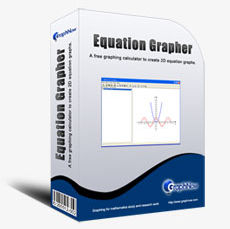Write a comment!

 Program info:Operating systems: WinXPInstallation: Install and UninstallLanguages: EnglishRelease status: New Release Popular programsMath Ninja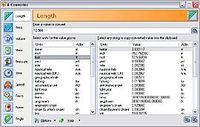A-Converter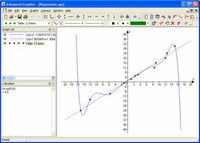Advanced Grapher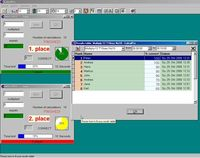CalculPro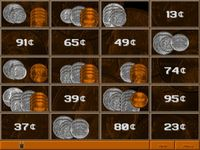Animated Money